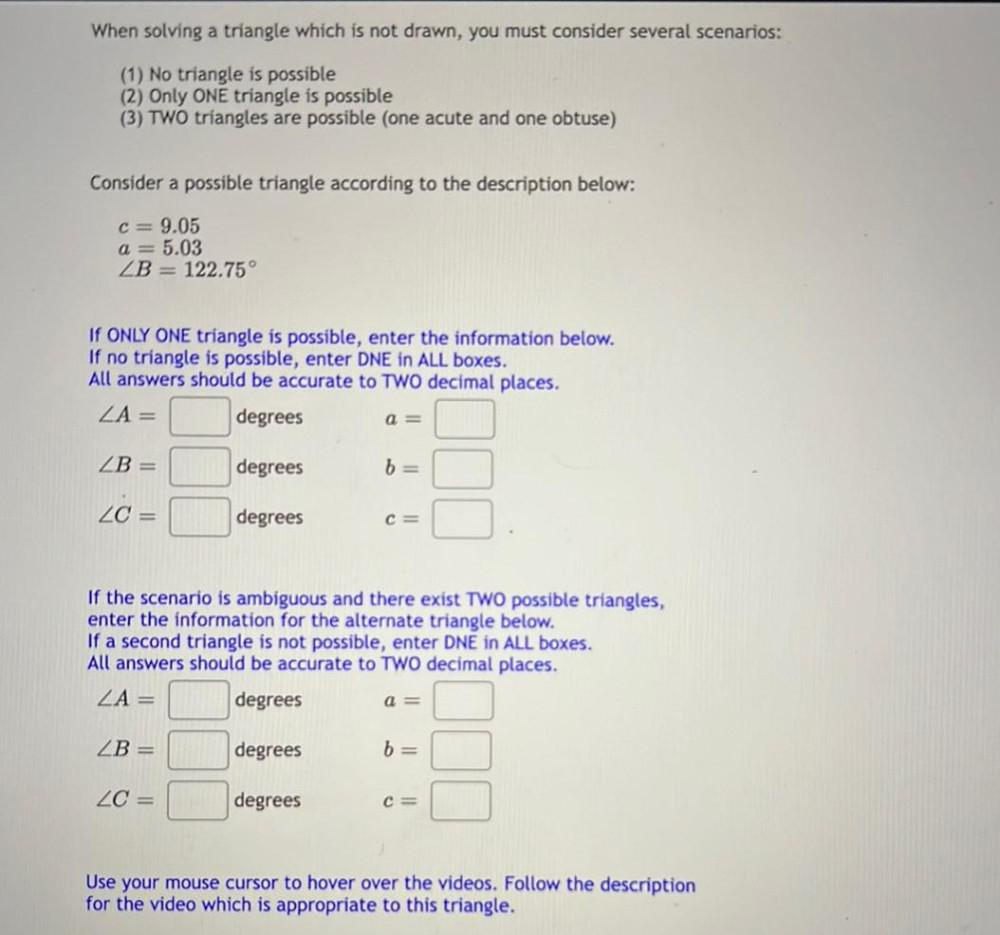Question:

# When solving a triangle which is not drawn, you must consider several scenarios: (1) No triangle is possible (2) Only ONE triangWhen solving a triangle which is not drawn, you must consider several scenarios: (1) No triangle is possible (2) Only ONE triangle is possible (3) TWO triangles are possible (one acute and one obtuse) Consider a possible triangle according to the description below: C= 9.05 a = 5.03 ZB = 122.75° If ONLY ONE triangle is possible, enter the information below. If no triangle is possible, enter DNE in ALL boxes. All answers should be accurate to TWO decimal places. ZA= degrees a = ZB = degrees b= ZC = degrees C If the scenario is ambiguous and there exist TWO possible triangles, enter the information for the alternate triangle below. If a second triangle is not possible, enter DNE in ALL boxes. All answers should be accurate to TWO decimal places. ZA= degrees a = ZB = degrees b= ZC = degrees C= Use your mouse cursor to hover over the videos. Follow the description for the video which is appropriate to this triangle.